How Cheenta works to ensure student success?
Explore the Back-Story

# ISI Entrance 2010 - B.Math Subjective Paper| Problems & SolutionsHere, you will find all the questions of ISI Entrance Paper 2010 from Indian Statistical Institute's B. Math Entrance. You will also get the solutions soon of all the previous year problems.

Problem 1:

Prove that in each year, the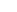th day of some month occurs on a Friday.

Problem 2:

In the accompanying figure,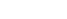is the graph of a one-to-one continuous function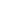. At each point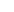on the graph of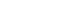, assume that the areas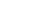and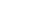are equal. Here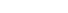are the horizontal and vertical segments. Determine the function.

Problem 3:

Show that. for any positive integer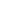the sum of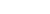consecutive positive integers cannot be a perfect square.

Problem 4:

If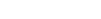satisfy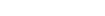prove that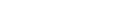.

Problem 5:

Let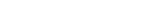be positive real numbers. Compute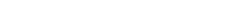.

Problem 6:

Let each of the vertices of a regular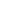-gon (polygon ofequal sides and equal angles) be coloured black or white.
(a) Show that there are two adjacent vertices of the same colour.
(b) Show there are 3 vertices of the same colour forming an isosceles triangle.

Problem 7:

Let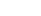be real numbers and. assume that all the roots of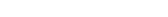have the same absolute value, Show thatif, and only if,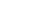.

Problem 8:

I etbe a real-valued differentiable function on the real line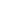such that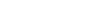exists, and is finite. Prove that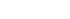.

Problem 9:

Let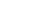be a polynomial with integer coefficients. Assume that 3 divides the value f(n) for each integer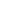. Prove that whenis divided by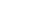the remainder is of the form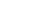, where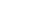is a polynomial with integer coefficients.

Problem 10:

Consider a regular heptagon (polygon of 7 equal sides and equal angles) ABCDEFG.

(a) Prove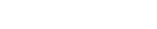.
(b) Using (a) or otherwise, show that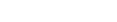. (See the figure appearing in the next page.)

Here, you will find all the questions of ISI Entrance Paper 2010 from Indian Statistical Institute's B. Math Entrance. You will also get the solutions soon of all the previous year problems.

Problem 1:

Prove that in each year, theth day of some month occurs on a Friday.

Problem 2:

In the accompanying figure,is the graph of a one-to-one continuous function. At each pointon the graph of, assume that the areasandare equal. Hereare the horizontal and vertical segments. Determine the function.

Problem 3:

Show that. for any positive integerthe sum ofconsecutive positive integers cannot be a perfect square.

Problem 4:

Ifsatisfyprove that.

Problem 5:

Letbe positive real numbers. Compute.

Problem 6:

Let each of the vertices of a regular-gon (polygon ofequal sides and equal angles) be coloured black or white.
(a) Show that there are two adjacent vertices of the same colour.
(b) Show there are 3 vertices of the same colour forming an isosceles triangle.

Problem 7:

Letbe real numbers and. assume that all the roots ofhave the same absolute value, Show thatif, and only if,.

Problem 8:

I etbe a real-valued differentiable function on the real linesuch thatexists, and is finite. Prove that.

Problem 9:

Letbe a polynomial with integer coefficients. Assume that 3 divides the value f(n) for each integer. Prove that whenis divided bythe remainder is of the form, whereis a polynomial with integer coefficients.

Problem 10:

Consider a regular heptagon (polygon of 7 equal sides and equal angles) ABCDEFG.

(a) Prove.
(b) Using (a) or otherwise, show that. (See the figure appearing in the next page.)

This site uses Akismet to reduce spam. Learn how your comment data is processed.

### Knowledge Partner